Perpendicular to a line through a point on it

Chapter 14 Class 6 Practical Geometry
Concept wise

Let’s take an example

1. Given a line l
with point P marked on it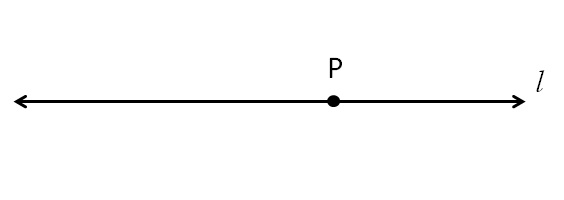2. Put ruler on the line so that line is exactly above the ruler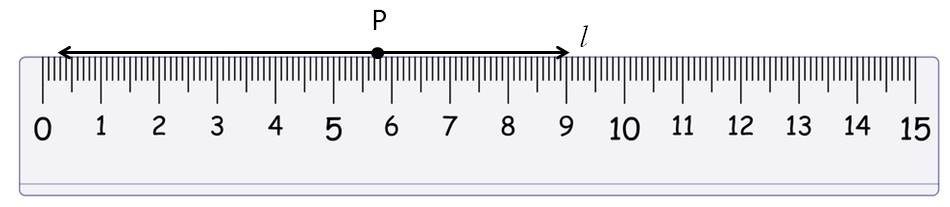3. Place set-square on top of the scale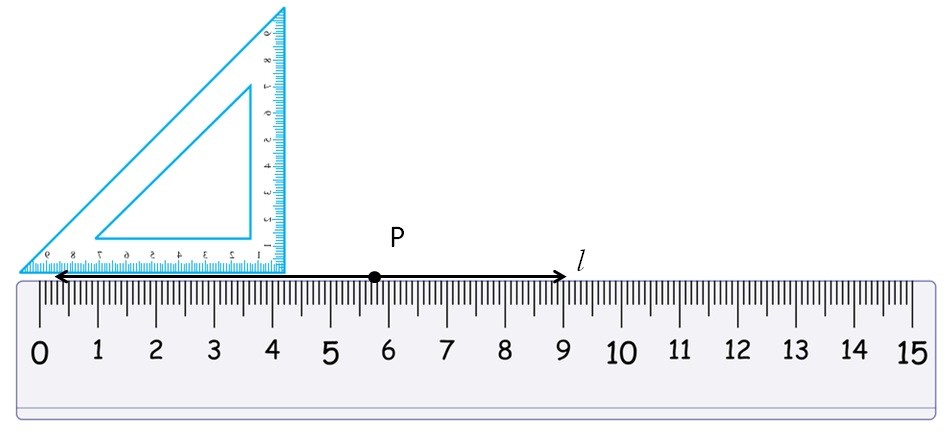1. Move set square so that its edge is at the point P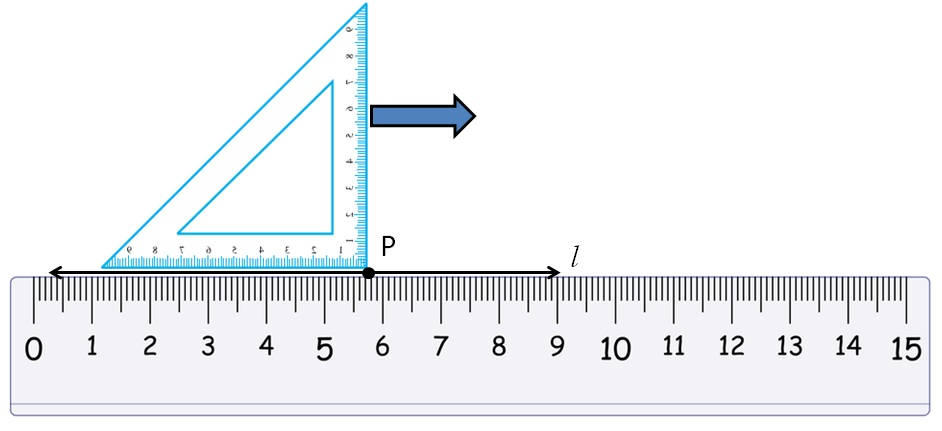2. Draw a line along the edge of the set square, keeping set-square firm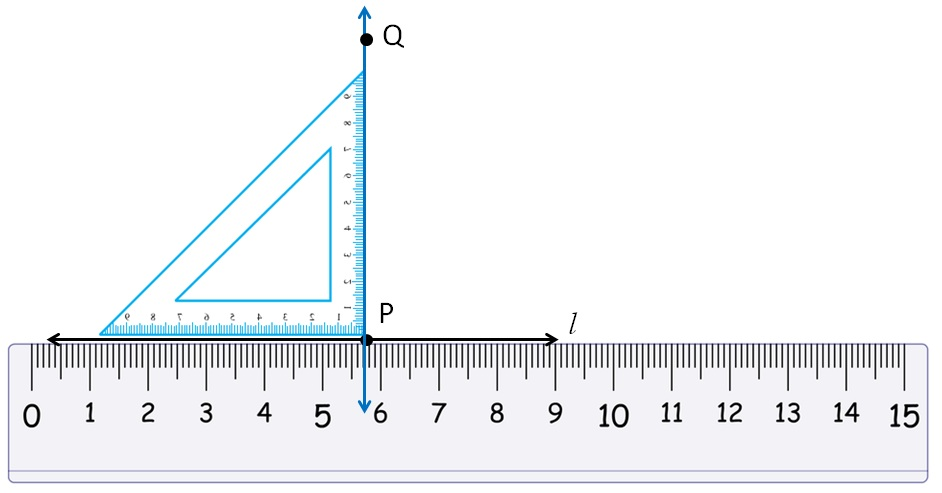So, PQ is the line perpendicular to l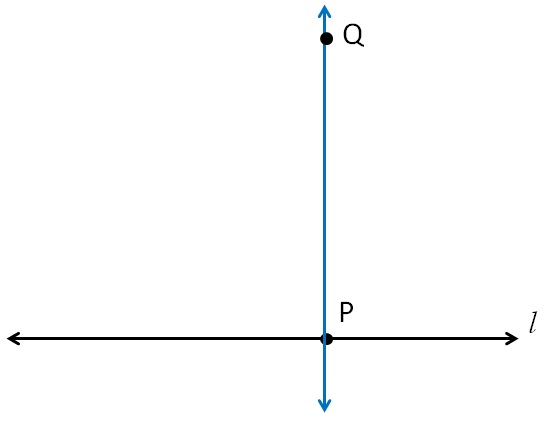Get live Maths 1-on-1 Classs - Class 6 to 12